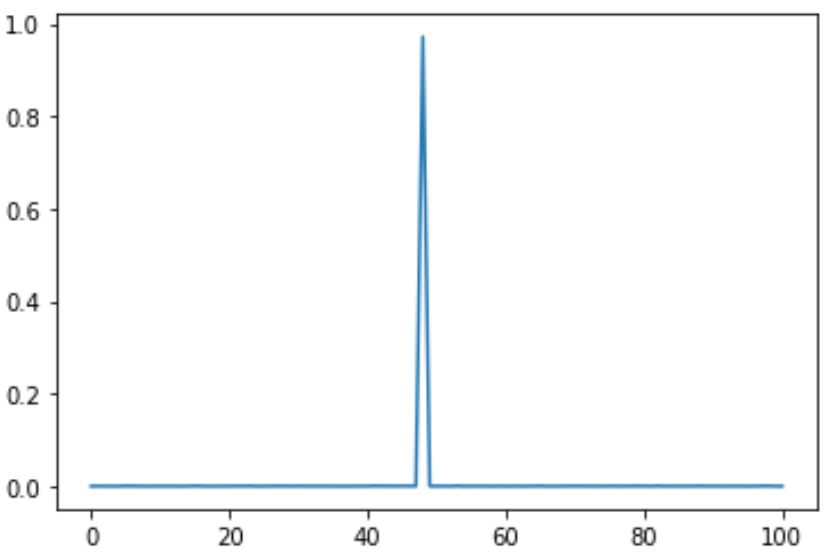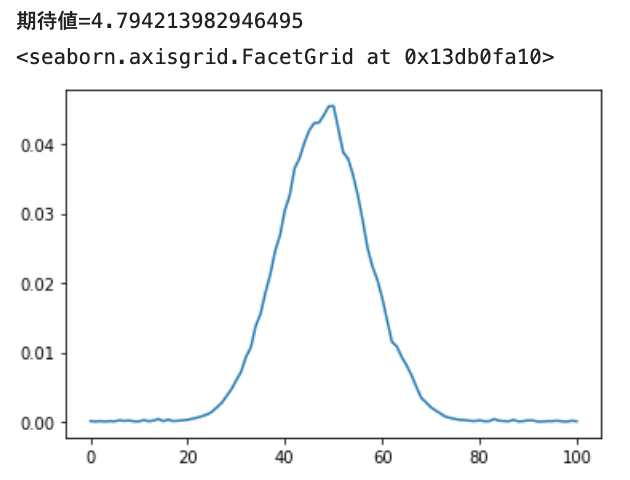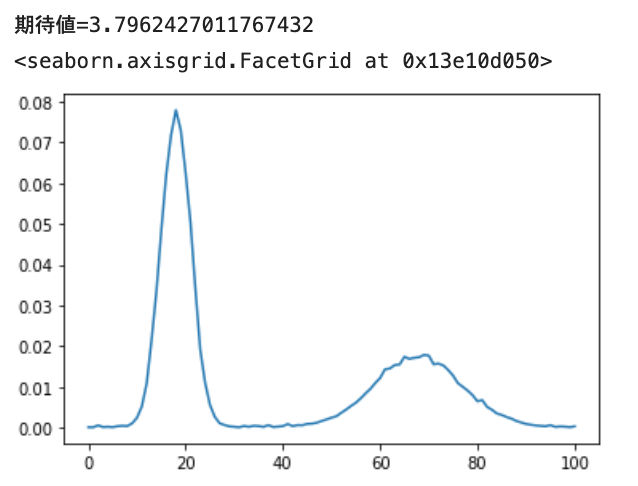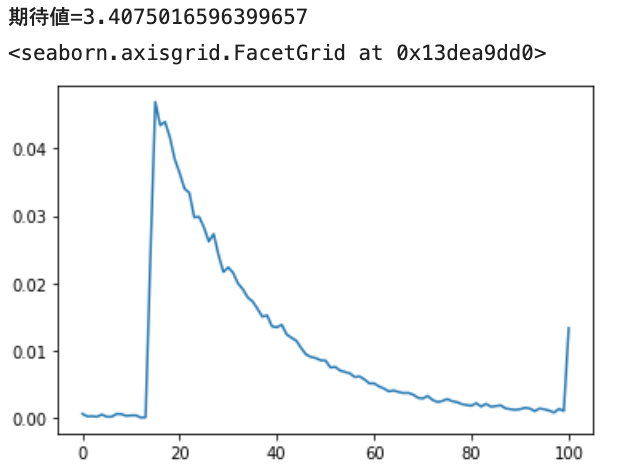# Introduction

The other day, when I was looking at the implementation of muzero-general, there was an interesting way to categorically predict continuous values.

If you think about it carefully, I thought that it would be possible to express the prediction of an arbitrary probability distribution of continuous values, and when I verified it, it seemed to work, so I'll make a note of it.

# Predict continuous values ​​categorically

If you use MeanSquaredError to predict continuous values, you basically assume that the distribution of the values ​​you want to predict is a normal distribution (and you are only interested in the expected values). So, for example, if the distribution has two mountains, it may be a little inconvenient to predict the distance between them. I don't know how wide it is (dispersion, etc.). If you have a fixed range of values ​​(of interest), you can do the following:

For example, if the range of values ​​is 0 to 10, then you decide on a point with a value of 11 such as `v = [0, 1, 2, ..., 10]`. First, set `p [0 ~ 10] = 0`, For example, the value 3.7 is expressed as p  = 0.3, p  = 0.7. If it is 0.1, then p  = 0.9 and p  = 0.1. If it is 3, p  = 1.0. In short, it feels like allocating the degree of belonging to both ends of the value.

Conversely, if you want to calculate the original value from this p, calculate the expected value `sum (p * v)`.

If you write it in

code, it will look like this.

``````import numpy as np

SUPPORT_SIZE = 11
VALUE_RANGE = [0., 10.]

def scalar_to_support(scalars):
values = np.array(scalars)
min_v, max_v = VALUE_RANGE
values = np.clip((values - min_v) / (max_v - min_v), 0., 1.)
key_values = np.linspace(0., 1., SUPPORT_SIZE)

r_index = np.searchsorted(key_values, values, side="left")  # a[i-1] < x <= a[i]
l_index = np.clip(r_index-1, 0, len(key_values))
left_vs = key_values[l_index]
right_vs = key_values[r_index]

interval = key_values - key_values
left_ps = 1-(values - left_vs)/interval
right_ps = 1-(right_vs - values)/interval

vectors = np.zeros((len(values), SUPPORT_SIZE))
for i in range(len(scalars)):
vectors[i, l_index[i]] = left_ps[i]
vectors[i, r_index[i]] = right_ps[i]

return vectors

def support_to_scalar(supports):
min_v, max_v = VALUE_RANGE
key_values = np.linspace(min_v, max_v, SUPPORT_SIZE)
supports /= supports.sum(axis=1, keepdims=True)
return np.sum(supports * key_values, axis=1)
``````
• In the implementation of muzero, an interesting conversion is performed as a pre-processing, but it is omitted.

For training, the output should be softmax (11 elements in this case) and the Loss function should be CrossEntropy.

# Verification of whether the probability distribution can be learned

Let's verify it using the PyTorch that we just learned.

## Prediction of constants

First, let's see if we can predict one value in a fixed manner.

code
``````# on jupyter notebook
import torch
import torch.nn.functional as F
import matplotlib.pyplot as plt

SUPPORT_SIZE = 101

class Net(torch.nn.Module):
def __init__(self):
super().__init__()
self.fc = torch.nn.Linear(1, SUPPORT_SIZE)

def forward(self, x):
x = self.fc(x)
x = F.softmax(x, dim=1)
return x

def get_dummy_input(batch_size):
dummy_inputs = np.random.random((batch_size, 1)).astype("float32")

def constant_target_value_fn(const):
def fn(batch_size):
return [const] * batch_size
return fn

def train_model(model, target_value_fn, epoch=1000, lr=0.01, batch_size=16):

loss_history = []
for ep in range(epoch):
target_values = target_value_fn(batch_size)
target_supports = torch.tensor(scalar_to_support(target_values))
#
outputs = model(get_dummy_input(batch_size))
losses = torch.mean(- target_supports * torch.log(outputs))
losses.backward()
optimizer.step()
loss_history.append(losses.item())

plt.plot(loss_history)
plt.show()

#######################

model = Net()
train_model(model, constant_target_value_fn(4.8))
outputs = model(get_dummy_input(5)).detach().numpy()
print(f"Expected value={np.mean(support_to_scalar(outputs))}")

vs = np.mean(outputs, axis=0)
plt.plot(vs)
``````

I set the target range to 0 to 10 and the size of the output vector to 101, and predicted a constant of 4.8. The prediction result is like this. There seems to be no problem.

`Expected value = 4.840604254500373`• The horizontal axis is 0 to 100, but please think that it is 0 to 10. The same applies thereafter.

## Prediction of normal distribution

Next, let's train the normal distribution (loc = 4.8, scale = 0.9).

code
``````from scipy import stats

def norm_fn(loc, scale):
def fn(batch_size):
dist = stats.norm(loc=loc, scale=scale)
return dist.rvs(batch_size)
return fn

model = Net()
distribution_fn = norm_fn(4.8, 0.9)
train_model(model, distribution_fn, epoch=1000, batch_size=1024)
outputs = model(get_dummy_input(5)).detach().numpy()
print(f"Expected value={np.mean(support_to_scalar(outputs))}")

vs = np.mean(outputs, axis=0)
plt.plot(vs)

``````Good vibes.

## Prediction of mixed normal distribution

Next, let's learn two normal distributions, Normal (loc = 1.8, scale = 0.3, probability = 0.6) and Normal (loc = 6.8, scale = 0.9, probability = 0.4).

code
``````from collections import Counter
def multi_norm_fn(loc_scale_prob_list):
def fn(batch_size):
values = []
for loc, scale, prob in loc_scale_prob_list:
dist = stats.norm(loc=loc, scale=scale)
values.append(dist.rvs(batch_size))
ps = np.array([p for _, _, p in loc_scale_prob_list])
ps = ps / np.sum(ps)
count = Counter(np.random.choice(range(len(values)), size=batch_size, p=ps))
ret = []
for i, cnt in count.items():
ret += list(values[i][:cnt])
return ret
return fn

model = Net()
distribution_fn = multi_norm_fn([
[1.8, 0.3, 0.6],
[6.8, 0.9, 0.4],
])
train_model(model, distribution_fn, epoch=1000, batch_size=1024)
outputs = model(get_dummy_input(5)).detach().numpy()
print(f"Expected value={np.mean(support_to_scalar(outputs))}")

vs = np.mean(outputs, axis=0)
plt.plot(vs)

``````Oh, you can do two mountains properly. Also, the size of the base is expressed.

## Exponential distribution prediction

Finally, there is the exponential distribution (loc = 1.4, scale = 2.0).

• The normal exponential distribution (2) is translated to the right by 1.4.
code
``````def exp_fn(loc, scale):
def fn(batch_size):
dist = stats.expon(loc, scale)
return dist.rvs(batch_size)
return fn

model = Net()
distribution_fn = exp_fn(1.4, 2.0)
train_model(model, distribution_fn, epoch=1000, batch_size=1024)
outputs = model(get_dummy_input(5)).detach().numpy()
print(f"Expected value={np.mean(support_to_scalar(outputs))}")

vs = np.mean(outputs, axis=0)
plt.plot(vs)
``````It is expressed that the peak rises from around 1.4 and gradually falls. It also shows that there are some values ​​above 10.

## Digression

Also, I think it's quite perfect for predicting continuous values ​​that circulate like angles. It seems that 10 degrees and 350 degrees are actually close to each other with a little ingenuity.

# at the end

So, I was able to confirm that continuous value prediction is OK with Cross Entropy in Categorical. This kind of technique may be mentioned in recent books, but I personally made a note of it because it was new.